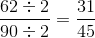ISEE Middle Level Math : How to add fractions

Example Questions

Example Question #47 : How To Add Fractions

Add: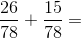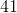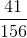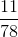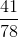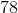Explanation:

To solve, add the numerators and leave the denominators the same: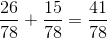Answer:Example Question #48 : How To Add Fractions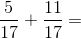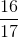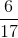Explanation:

Add the numerators and keep the denominator the same: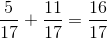Answer:Example Question #31 : How To Add Fractions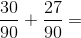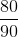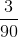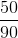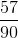Explanation:

Add the numerators and keep the denominator the same: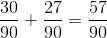Answer:Example Question #50 : How To Add Fractions

Solve the equation.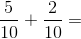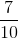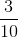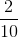Explanation:

When adding fractions that have the same denominator, only the numerators are added together. Add the numerators and keep the denominators: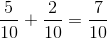Answer:Example Question #32 : How To Add Fractions

Solve the equation: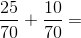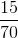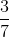Explanation:

When adding fractions that have the same denominator, only the numerators are added together. Add the numerators and keep the denominators: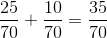This fraction can be reduced by dividing both the numerator and denominator by a common factor.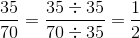Answer:Example Question #431 : Arithmetic

Solve the equation: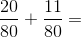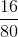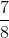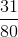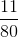Explanation:

When adding fractions that have the same denominator, only the numerators are added together. Add the numerators and keep the denominators: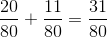Answer:Example Question #432 : Arithmetic

Solve the equation: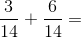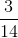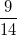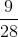Explanation:

When adding fractions that have the same denominator, add the numerators only. To solve add the numerators and keep the denominators the same: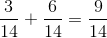Answer:Example Question #433 : Arithmetic

Solve the equation: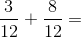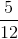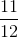Explanation:

When adding fractions that have the same denominator, only the top numbers are summed. Add the numerators and leave the denominators the same: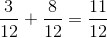Answer:Example Question #434 : Arithmetic

Solve the equation: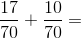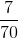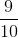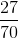Explanation:

When adding fractions that have the same denominator, only the top numbers are summed. Add the numerators and leave the denominators the same: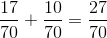Answer:Example Question #435 : Arithmetic

Add: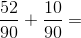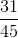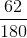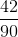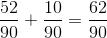Reduce the fraction: